# Quality factor

This discussion is new for January 2017, and is just a taste of what quality factor is all about. For now we provide a non-microwave example, and some discussion of transmission line Q.

Quality factor, or simply, Q, is a unit-less figure of merit for passive structures: a measurement of stored energy divided by dissipated energy. Components such as capacitors, inductors, filters, resonant cavities, even transmission lines have quality factors associated with them. The higher the Q, the better your career!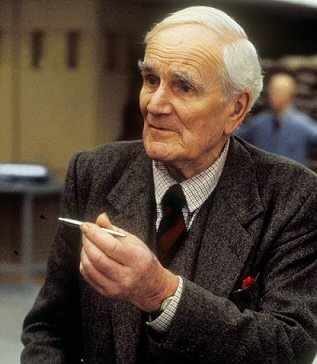Welsh actor Desmond Llewelyn played Q in 17 James Bond movies.
Bond - "They always said the pen was mightier than the sword."
Q - "Thanks to me they were right!"

Read more Q quotes on jamesbondwiki.com.

## A non-microwave example of quality factor

If you consider a swing set, the energy stored is the mass of the kid that is riding it, times the height difference he/she moves. The lost energy is a mixture of air resistance and friction in the chain or cable, particularly at the top link.  How do you increase the Q?  You increase the mass to store more energy, and increase the length of the chain, which slows the period of oscillation and thereby decreases air resistance. If that is non-intuitive for you, consider a job as a sales representative, you will get a nice expense account.

A famous French experimenter, Léon Foucault, made such a high-Q "swing set" back in 1851 in Paris France.  In doing so he added visual proof that the Earth rotates once every 24 hours, by showing that the pendulum's plane of oscillation rotated 360 degrees in 32.7 hours. You would not be alone if you thought it would rotate 360 degrees each day, however, that only happens on the poles of the earth, because the angular frequency is reduced by sine of the latitude.  Read more on Wikipedia. Thus, you can measure your latitude with Foucault's pendulum.  Foucault is connected to one of our Microwave Hall-of-Famers, Hippolyte Fizeau. Between the two of them they master-minded the first terrestrial measurement of the speed of light.  Foucault did NOT have a college degree, but his name is one of 72 inscribed on the base of the Eiffel Tower.

The video below is a re-creation of the original 28 kilogram bob and 67-meter cable pendulum, as the original bob smashed into the floor when its cable broke in year 2010.  ~160 years is a pretty good run. A good homework assignment for high school physics class would be to calculate the length of the cable based on the period of oscillation in the video below...

Foucault's pendulum at Musée des Arts et Métiers, Paris France

## Q in transmission lines: an example

The Q of a transmission line is defined as:

Q=β/(2α)

Where beta is the phase constant and alpha is the attenuation constant of the overall wave propagation constant, gamma.  For more discussion of the propagation constant, look here. For a derivation of Q for a transmission line, check out Pozar. So, what does Q look like for a real life example?

How about we look at stripline using 254um Duroid, with ER=2.2 and tanD =0.0005, and 30um copper metalization, but take some spreadsheet shortcuts rather than whip out \$50K worth of EDA software?

Wavelength is frequency/velocity, and velocity in this case is the speed of light (300 mm/nanosecond) divided by the square-root of the dielectric constant in this TEM example, or ~202 millimeters/nanosecond in this case. At 10 GHz, wavelength is ~20mm. Aren't you glad we didn't use inches and feet here?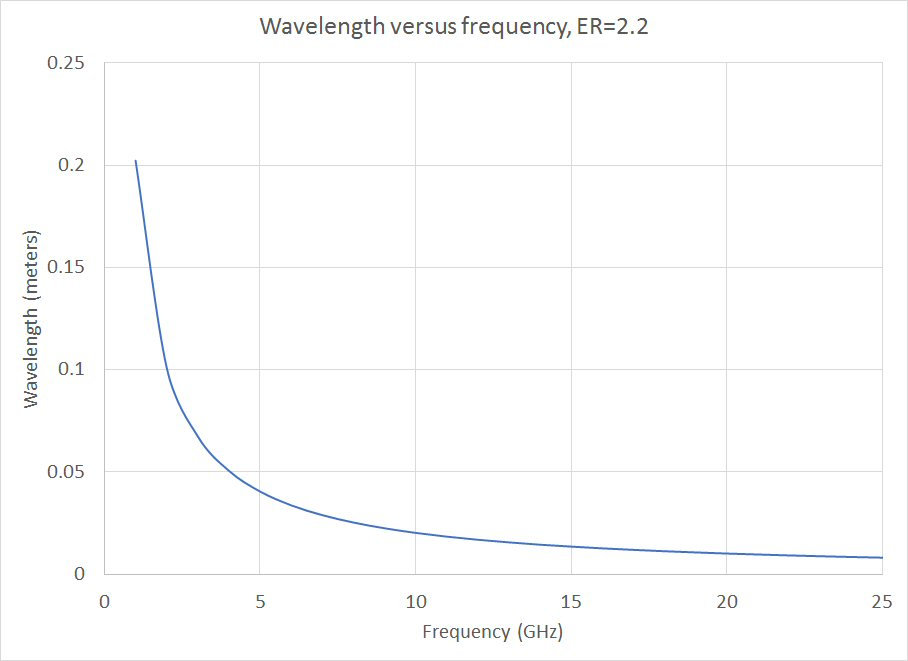Beta is 2π/wavelength, you should remember that. At 10 GHz, ~300 radians of phase are observed along one meter of this transmission line.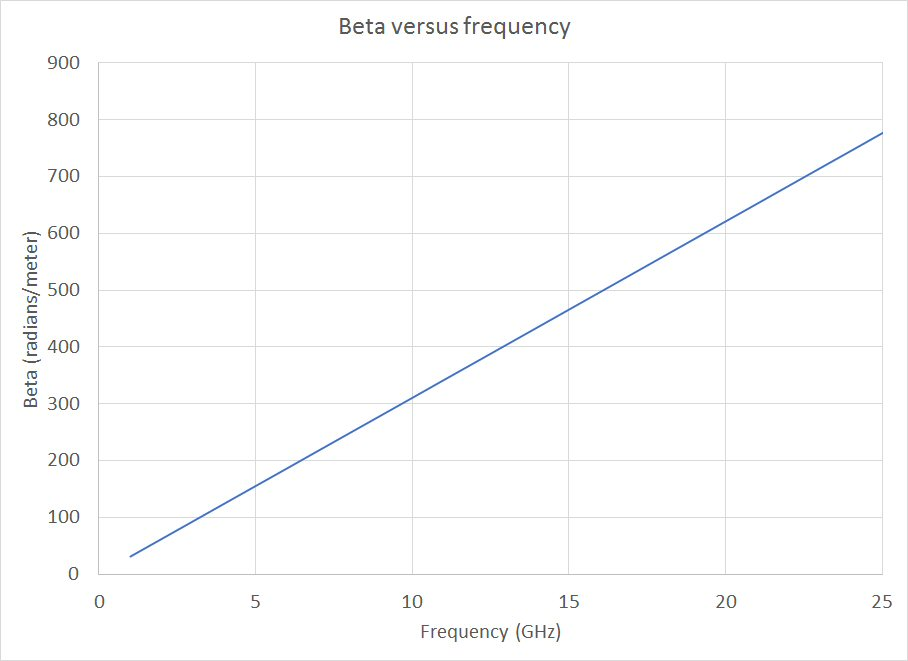Alpha has two components worth considering: metal loss and dielectric loss. Dielectric loss is proportional to frequency, while metal loss is proportional to square-root frequency. AlphaD is calculated as:

Where lambda is the wavelength in the media that we plotted above. Hold on a second while we compute alphaC and we'll plot them together...

AlphaC (loss due top metal) is:

alphaC=R'/(2Z0)

R' is the series resistance per unit length, and depends on metal conductivity and skin depth.  In our case, the conductor strip is ~350um wide (using 30um thickness for 50 ohms), according to our stripline calculator, for 50 ohms.  As an approximation, let's consider that the two surfaces (top and bottom) conduct equally, we will ignore the conduction on the 30 micron edges, and observe that the skin depth is much less than the strip thickness.

RF sheet resistance must be calculated as an intermediate step toward R'.  Sheet resistance goes up with frequency, due to skin depth decreasing.

RRFSR=[(pi*μ0μRf)/2σ]^0.5

Where σ is the metal conductivity (6E7 S/m for copper) and μ0μR are the permeability terms (μR is 1 for copper and can be disregarded). At 10 GHz we are looking at 0.026 ohms/square.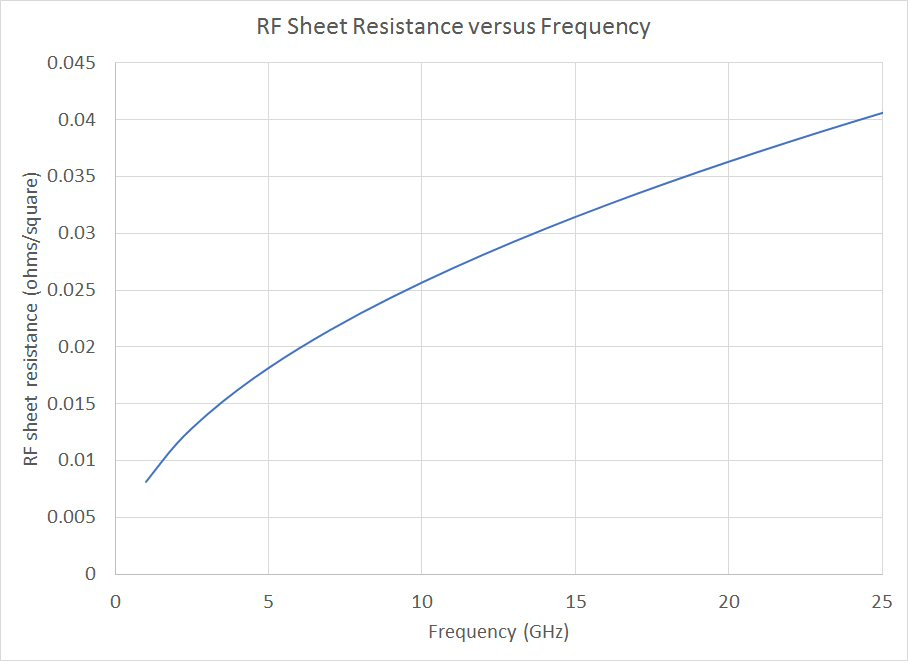Say, why aren't we making equation graphics on this page?  Because it is a pain in the ass to take equations from Microsoft Word, dump them into Paint Shop Pro, and try to store them in a format that is legible.  Maybe we'll go back and fix this later. Maybe Microsoft will make a seamless interface in the meantime, but don't hold your breath either way.

Now we calculate alphaC and plot it on top of alphaD.  R' is the RF sheet resistance applied to a meter in length, reduced by half because there are two conducting surfaces.

alphaC=R'/2Z0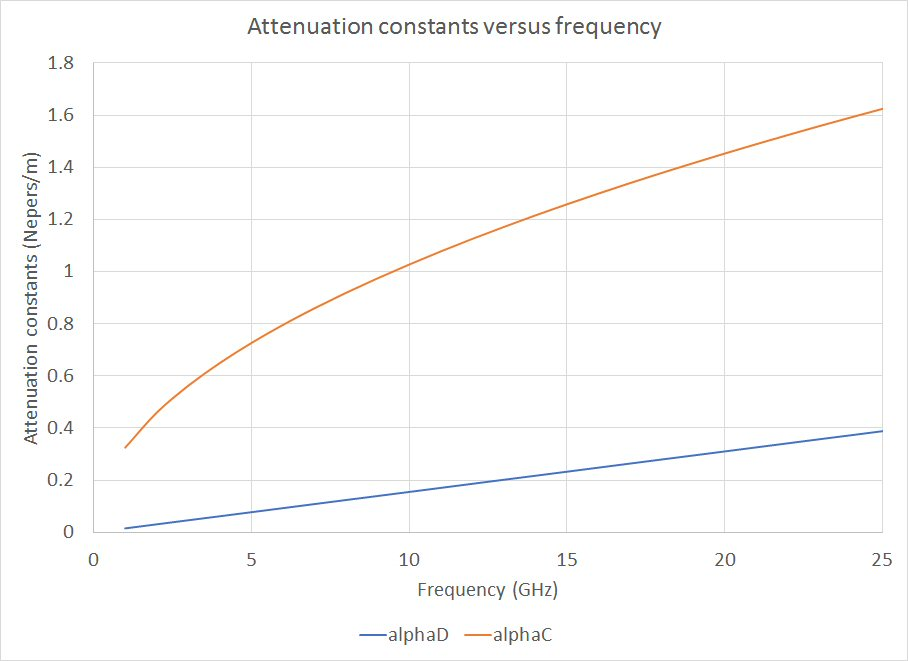You will observe that alphaD is a straight line, while alphaC is curving at square-root of frequency.  If you held a horse race to 200 GHz, alphaD always comes out on top, like Aesop's tortoise and the hare. But at low frequency, for a good dielectric, metal loss is always the bigger culprit. The picture below is from Wikipedia.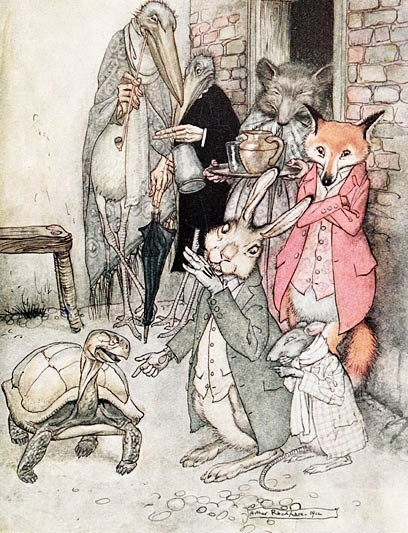Dielectric tortoise versus metal hare

Its time to plot Q for our example: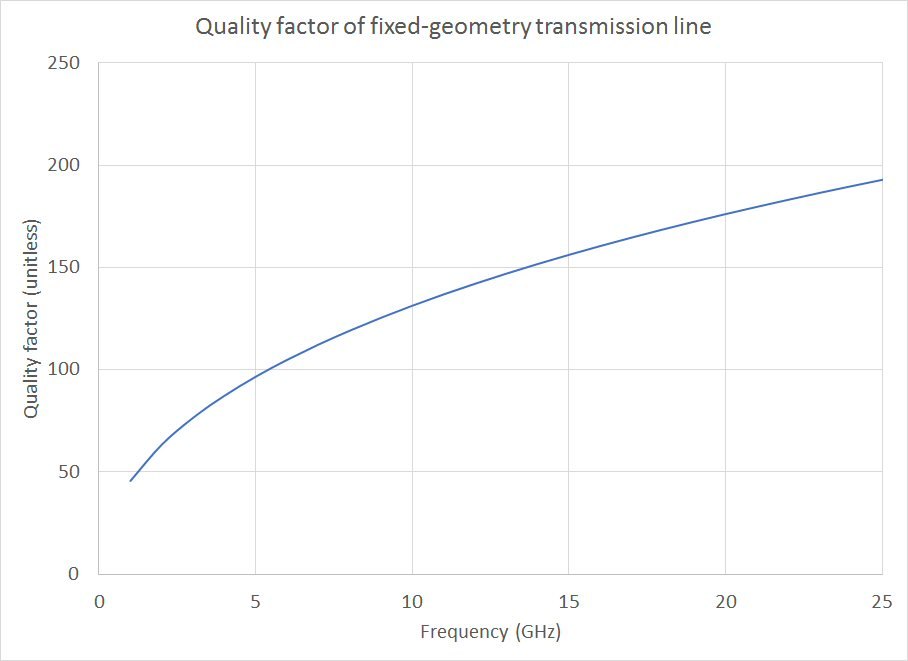What's going on here, Q actually increases with frequency?  Yes, in this example it does, as we assumed that the stripline geometry is the same for all frequencies.  You might observe this effect if you designed Wilkinson power dividers on 100um GaAs at 10 GHz and 35 GHz. At 35 GHz you should see less loss than at 10 GHz; at higher frequencies all bets are off as the substrate will start to mode and radiation losses will take their toll.

Speaking of moding, something to consider is the maximum Q that you can get with a transmission line that can still carry your wave mode-free.  In this case, the height of the substrate will have to decrease with frequency, and so will the conductor with, and so will Q. Below, we scaled Q, assuming that the 508um substrate can only support 10 GHz. Q is dropping nearly as the square-root of frequency in this case, and it would be exactly square-root function if not for dielectric loss.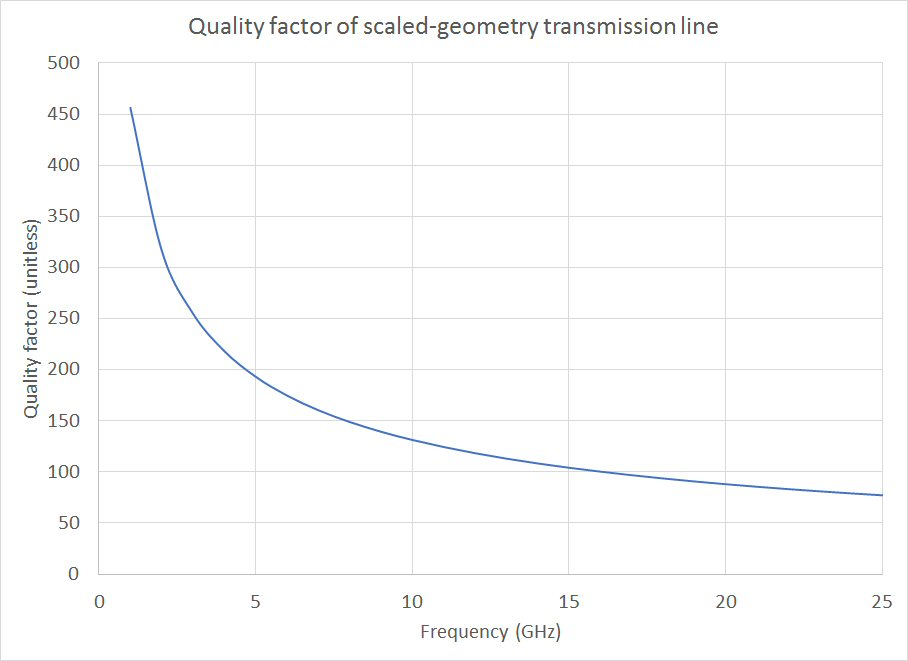What's missing from this discussion (and we will add later): how do you calculate Q from measure S-parameters of a transmission line?

## Q in inductors and capacitors

Quality factor in a capacitor is expressed as:

QCap=|XC|/ESR

Where ESR is its equivalent series resistance.

Similarly, quality factor in a inductor is expressed as:

QInd=|XL|/ESR

Where ESR is its equivalent series resistance.

## Q in filters

Quality factor is generally the inverse of bandwidth: High Q means you can achieve tighter bandwidths in band-pass filters. Come back soon...

Author : Unknown Editor